# Eye Level Math Online

Eye Level Math helps improve problem-solving skills by enabling
students to master concepts through a small-step approach.

##### GET YOUR FREE TRIAL NOW!

Eye Level Blended Learning gives you the power and flexibility to master your learning. Sign up to try out Eye Level Blended Learning for free trial at no extra cost.

#### Online Math Programs

Eye Level Math Online is a unique online program built to help students improve their calculation ability through mastery learning from virtually anywhere.##### Level 3 | Booklet 1 | Part 1 Understanding Numbers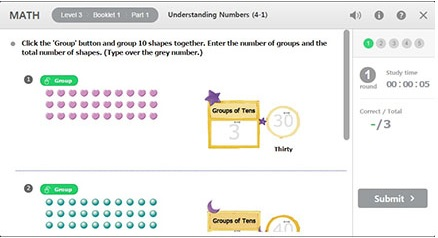##### Level 7 | Booklet 6 | Part 2 Addition of 1-Digit Numbers to 10~19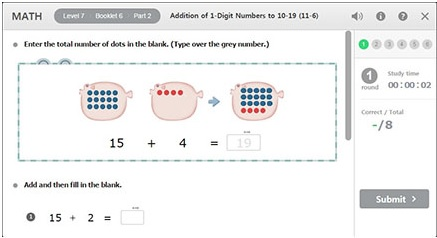##### Level 10 | Booklet 8 | Part 2 Addition of 2-Digit Numbers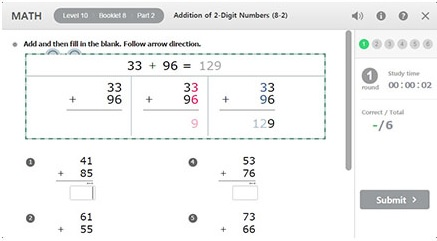##### Level 12 | Booklet 6 | Part 1 Multiplication Facts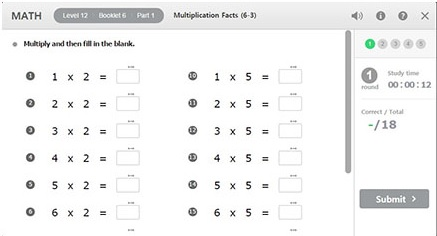##### Level 15 | Booklet 1 | Part 1 Division of 3-Digit Numbers by 1-Digit Numbers#### Development of Mathematical Thinking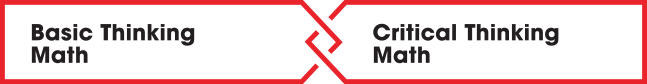Build a basic Foundation
Of mathematics.

Basic Thinking Math enables students to
complete the foundation of mathematics and
covers the following study areas: Numbers,
Arithmetic, Measurement, and Equations.

and Applications.

Critical Thinking Math enables students to
develop depth perception, problem solving,
reasoning skills, and covers the following study
areas: Patterns and Relationships, Geometry,
Measurement, Problem Solving, and Reasoning.

#### Mastery of Math Learning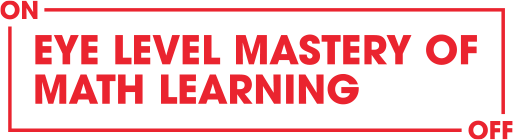##### ONLINE LEARNING

- Mastery learning with BTM repetition
- Maximization of motivation by online program
- Maximization of learning effect by using auto
scoring and instant feedback system
- Arithmetic game activity
- Easy access on accumulated records

##### OFFLINE LEARNING

- Simultaneous learning of BTM & CTM
- Learning of new concepts
- Improvement of problem solving skill with various
supplementary materials
- Well systemized assessment

#### Learning Materials

Numerical Figures Blocks and Shapes Clear Paper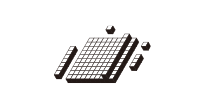Numerical Figures assist in developing the concepts of number and quantity.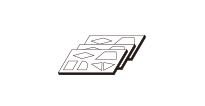Blocks and Shapes assist in developing depth perception by matching and finding the location of various shapes.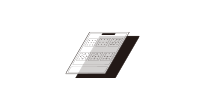Clear Paper is used to trace and copy various shapes. This helps students to develop their mental skills in relation to depth perception and location.

Colored Blocks Mirror Wooden Blocks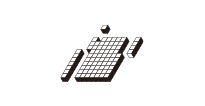Colored Blocks assist in the understanding of numbers as well as length. As students manipulate Colored Blocks, they develop their perception of depth and location.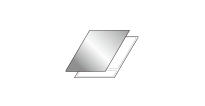Mirror activities require students to draw shapes symmetrically. This forms the basis for line symmetry that will be introduced in future studies.The manipulation of Wooden Blocks enables students to learn depth perception, location, and spatial relationships. This is crucial in the development of critical and analytical thinking skills.

Thinking Bricks Thinking Pentos Thinking Cubes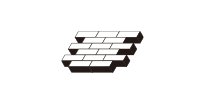Thinking Bricks consist of three cuboids that can be used to stack up the bricks. (5 pieces, 2 sets)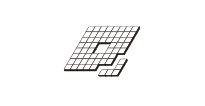Thinking Pentos are used to build a model built by putting the sides of unit squares together. (12 pieces)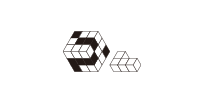Thinking Cubes are used to build a model that comprises the figures with concave surface built by putting together the faces of 3 or 4 cubes. (7 pieces)

#### About Eye Level Math Online Learning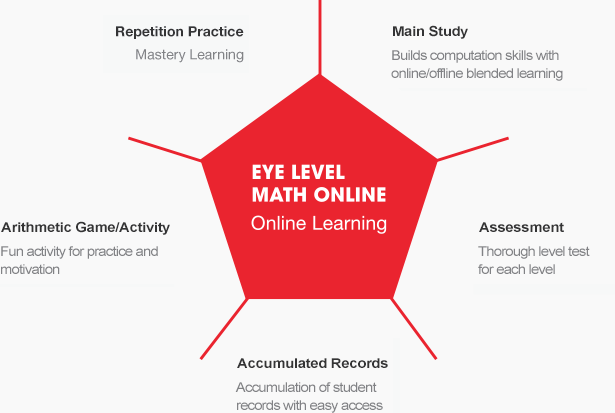#### Free Trial Information

##### Free Trial Information
###### Start your free trial now!

Try out this powerful learning program yourself: Eye Level Blended Learning gives you the power and flexibility to master your learning. Start the Eye Level Blended Learning for free trial at no extra cost.

##### Free Trial Period & Benefits
###### No Functional Limitations in Free Trial Program

There are no limitations to any Eye Level trial program routine. You can try out the program for 2 weeks as if you have paid for the entire program!

###### Progress Report & Instructor Comment

Progress Report created during the free trial period will be shared with the learning center you have selected for consultation. Our instructor will provide comments and advise you on your .strengths and weaknesses.

###### Receive Consultation at the Learning Center

Interested students & parents can visit the nearest Eye Level Center to set up a consultation. Eye Level instructors will recommend an appropriate learning progress based on each student’s “Eye Level” of learning pace.

##### Free Trial Includes
3 Children learn the numerical order of numbers up to 120 through expansion of numbers and by studying adding 1, 2, and 3. K4~K6
4 By studying how to add 1, 2, and 3, the numerical order up to 120 is understood. Children will also study how to subtract 1, 2, and 3.
5 Children are led to understand how to make numbers through the addition table, while developing their mental arithmetic skills. P1
6 This lesson develops the ability to perform mental arithmetic with addition facts. Practice enables children to mentally calculate and answer addition problems.
7 Based on learning the addition table, this lesson checks the complete understanding of (2-digit up to 19) + (1-digit) and further practices mental arithmetic to enable answering intuitively.
8 Study subtraction table. Understanding subtraction and developing mental calculation of subtraction.
9 Enables children to give intuitive answers by helping them understand the concept and method of (2-digit) – (1-digit). Completes the mental arithmetic of subtraction.
10 Using the mental arithmetic ability, students practice carrying out 2-digit addition with regrouping notations. Complete the study of written arithmetic of addition. P2
11 Using the mental arithmetic of subtraction ability, students practice 2-digit subtraction without borrowing notations. Complete the study of written arithmetic of subtraction.
12 Intensive practice with the times tables and introduction to basic multiplication P2
13 Based on the multiplication table, students understand the calculation theory and method of (2-digit) x (1-digit), and practice handling the regrouping in their head without written notations. Complete the study of multiplication.
14 Students become familiar with the division table and establish the basics of division. Students come to understand that the remainders should always be smaller than the divisor.
15 Completion of long division process. P3~P4